# Intermediate Geometry : How to find the slope of a tangent line

## Example Questions

### Example Question #1 : How To Find The Slope Of A Tangent Line

What is the slope of the tangent line to the graph of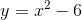when?Explanation:

To find the slope of the tangent line, first we must take the derivative of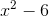, giving us. Next we simply plug in our given x-value, which in this case is. This leaves us with a slope of.

### Example Question #2 : How To Find The Slope Of A Tangent Line

Suppose the equation of a line is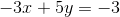.  What is the slope of the tangent line at?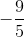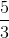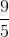Explanation:

Rewritein slope-intercept form,.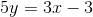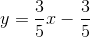The slope of the tangent line is.

### Example Question #3 : How To Find The Slope Of A Tangent Line

Suppose a function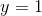.  What is the slope of the tangent line at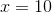?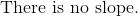Explanation:

Write the formula for slope-intercept form.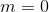The slope ofis always zero at every point on the domain.  Therefore, the slope atmust also be zero.